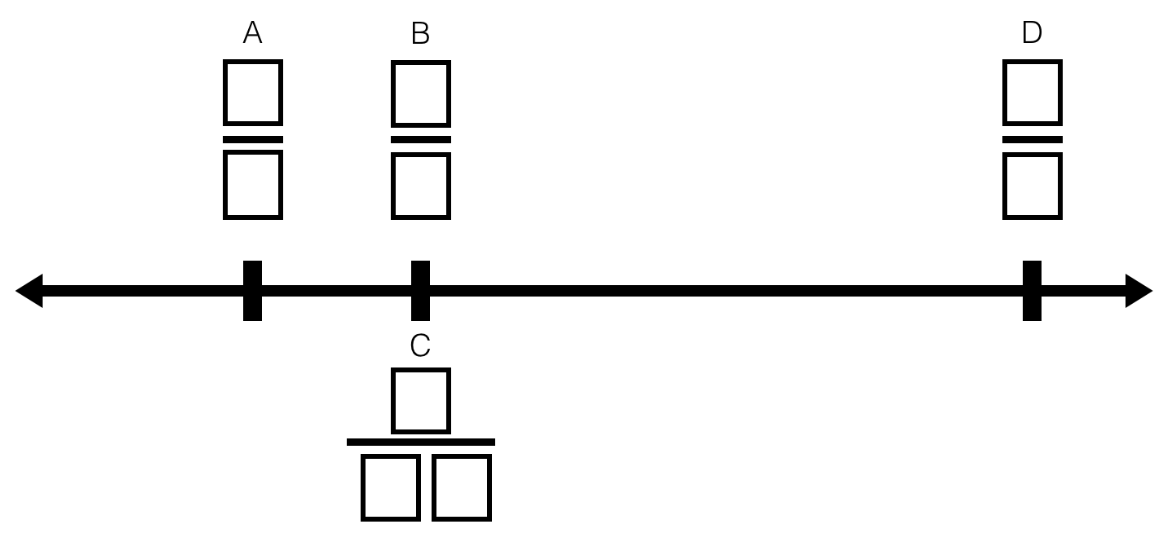# Comparing and Identifying Fractions on a Number Line

Directions: Using the digits 1 to 9 exactly once, place a digit in each box to create and place 4 fractions on the number line in the correct order. (fractions B & C are equal)### Hint

The sum of the equivalent fractions is greater than a half and greater than a quarter separate. How do you know if two fractions are equivalent? How do you know if fractions are less than or greater than another fraction?

There are many possible answers. Here are a couple: A = 1/7, B = 3/8, C = 9/24, D = 5/6; Another possibility is A = 1/5, B = 3/9, C = 8/24, D = 6/7; yet another possibility is A = 5/8, B = 3/4, C = 9/12 and D = 6/7. (or D could be 7/6)

Source: Graham FletcherBowen Kerins, and Kate Nowak

## Simple Patterns 2

Directions: Using the digits 1 to 9 at most one time each, place a digit …

1.Here is another answer a student found A = 5/8, B = 3/4, C = 9/12 and D = 6/7. (or D could be 7/6)

•2.B and C are not equivalent in your first possible answer. (3/6 > 9/27)

•Thank you for catching that Gina, the answer set has been updated.

3.This is so much fun! My students and I are wondering about A= 3/7, B=4/8, C=6/12 and D=9/5 and A= 2/7, B=3/6, C=9/18 and D=5/4 Thanks for sharing your work with us!

4.A group of students worked on this yesterday as they reviewed equivalent fractions and ordering…and they/we had so much fun doing! It was their first “open-middle” task with the recording sheet. I am so excited about this task. There were so many great discussions, mistakes, revisions…

5.Another possibility…I got 3/7, 2/4, 9/18, and 5/6.

6.My son spent a few hours seeking to find the most optimal answer based on the partitioning of the number line. He makes a case for 2/5, 3/6,7/14, 8/9. The difference or distance between 3/6 and 8/9 is approx. 4 times the distance between 2/5 and 3/6.

7.What fun. Today we were comparing fractions to 1/2. I wanted to test this out myself before I introduced it to the class. I thought, if I make B 1/2 or its equivalent, than I can use the left over digits to create a fraction less than 1/2, and another greater than 1/2. I came up with a = 3/9, b = 2/4 = 8/16, and d = 5/7. I wonder if any of my students will think this way.

8.I think a is 3/7, b is 2/4, d is 5/6, and c is 9/18.

9.I really like this problem and look forward to trying it out with my students. Is the placement on the line supposed to suggest some sort of scale or do the fractions just have to be A<B=C<D?

10.